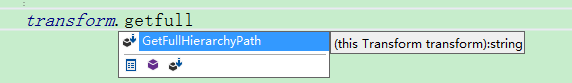using UnityEngine;

public static class TransformExtensions {
public static string GetFullHierarchyPath(this Transform transform) {
string path = "/" + transform.name;
while (transform.parent != null)
{
transform = transform.parent;
path = "/" + transform.name + path;
}
return path;
}
}

string path= transform.GetFullHierarchyPath();public static void SetParent(this Transform go, Transform par)
{
go.parent = par;
go.localPosition = Vector3.zero;
go.localEulerAngles = Vector3.zero;
}

effect.transform.SetParent(transform);